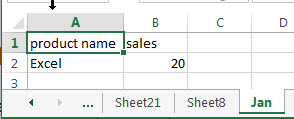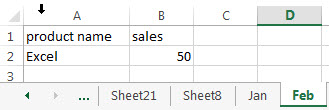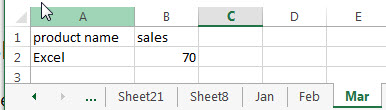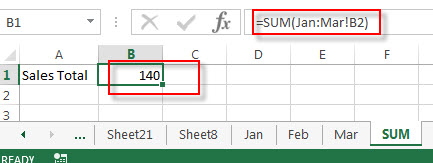# Sum Same Cell Range on Multiple Worksheets

This post will explains that how to use the Excel 3-D reference to reference the same cell or a range of cells on multiple worksheets, then try to sum the same cell range from multiple worksheet with an Excel formula.

The 3-D reference refers to the same cell or range on multiple sheets. And it is useful and convenient way to reference several worksheets that follow the same pattern or cells on each worksheet contain the same type of data.

## Sum Same Cell Range on Multiple Worksheets

Assuming that you have monthly sales report of Excel product in three different worksheets:If you want to sum identical cell range on multiple worksheets, you can create the following formula based on the SUM function:

`=SUM(Jan:Dec!B2)`

Type this formula in cell B1 under SUM worksheet.This formula contain one 3-D reference Jan:Dec!B2, and it will add cells B2 on worksheet Jan through Mar.

### Related Functions

• Excel SUM function
The Excel SUM function will adds all numbers in a range of cells and returns the sum of these values. You can add individual values, cell references or ranges in excel.The syntax of the SUM function is as below:= SUM(number1,[number2],…)…